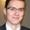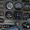## Converting decimals to fractions 1 (ex 3)

Title:
Converting decimals to fractions 1 (ex 3)
Description:

Converting decimals to fractions 1 (ex 3)

more » « less
Video Language:
English
Duration:
01:14Nikoleta Nikolaeva edited Bulgarian subtitles for Converting decimals to fractions 1 (ex 3)Nikoleta Nikolaeva edited Bulgarian subtitles for Converting decimals to fractions 1 (ex 3)Next Nhn edited Korean subtitles for Converting decimals to fractions 1 (ex 3)Next Nhn edited Korean subtitles for Converting decimals to fractions 1 (ex 3)Jiří Minarčík edited Czech subtitles for Converting decimals to fractions 1 (ex 3)Petra Jirůtková edited Czech subtitles for Converting decimals to fractions 1 (ex 3)Nina Nutsubidze edited Georgian subtitles for Converting decimals to fractions 1 (ex 3)Vera Dr edited Ukrainian subtitles for Converting decimals to fractions 1 (ex 3)